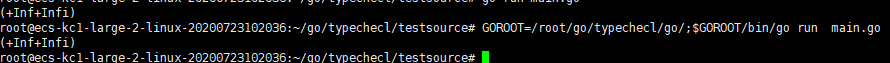{{ message }}

# cmd/compile: Dividing complex128 by zero does not report an error#41308

Closed
opened this issue Sep 10, 2020 · 3 comments
Closed

# cmd/compile: Dividing complex128 by zero does not report an error#41308

opened this issue Sep 10, 2020 · 3 comments
Labels

### What version of Go are you using (`go version`)?

```\$ go version
master
```

yes

### What did you do?

```package main

import (
"fmt"
)
func main() {
var x complex128 = complex(1, 2) // 1+2i
fmt.Println(x/ 0)
}```

an error

### What did you see instead?###ianlancetaylor commented Sep 10, 2020

 Please paste text as text, not as an image. Thanks. This is expected behavior. In floating point arithmetic, dividing a finite value by zero gives you an infinity, not an error. See "Floating-point operators" at https://golang.org/ref/spec#Arithmetic_operators.

###xiyichan commented Sep 10, 2020 • edited Loading

 ```package main import ( "testing" ) func TestConstInt(t *testing.T){ const x int =1 t.Log(x/0) } func TestVarInt(t *testing.T){ var x int =2 t.Log(x/0) } func TestVarFloat64(t *testing.T){ var x float64 =2.222 t.Log(x/0) } func TestConstFloat64(t *testing.T){ const x float64 =2.222 t.Log(x/0) } func TestConstComplex(t *testing.T){ const x complex128=complex(1,2) t.Log(x/0) } func TestVarComplex(t *testing.T){ var x complex128=complex(1,2) t.Log(x/0) }``` This is the test code I wrote. Why use const to modify float64 and complex will cause errors. @ianlancetaylor

###ianlancetaylor commented Sep 10, 2020

 In Go, constant arithmetic does not use the same rules as variable arithmetic. In particular, constant floating point values have no infinity, or NaN, or negative zero. See https://golang.org/ref/spec#Constant_expressions . In general for questions about the language it's better to use a forum. You will get better and faster answers. See https://golang.org/wiki/Questions.